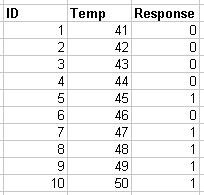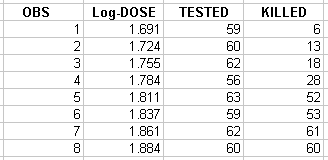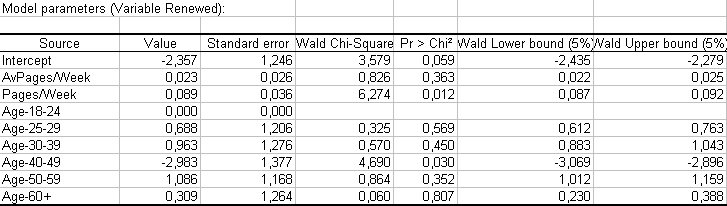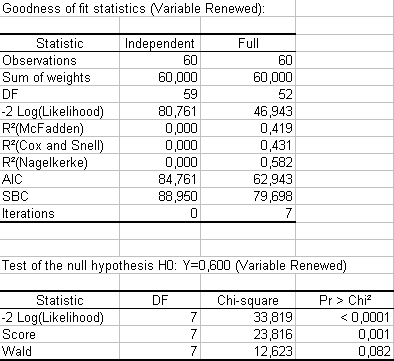Your data analysis solution

# Running a logistic regression in Excel

2018-01-30

This tutorial will help you set up and interpret a Logistic Regression in Excel using the XLSTAT software.
Not sure this is the modeling feature you are looking for? Check out this guide

## What is logistic regression?

Logistic regression, and related methods such as Probit analysis, are very useful techniques when one wants to understand or to predict the effect of a series of variables on a binary response variable (a variable which can take only two values, 0/1 or Yes/no, for example). Logistic regression can be helpful to model the effect of doses in medicine or agriculture, or to anticipate the likelihood of customers responding to a direct mail, or to evaluate the risk for a bank that a client will not pay back a loan.

With XLSTAT you can either run the logistic regression on raw data (the response is given as 0s and 1s) or on aggregated data (the response is a sum of "successes" or ones, and the number of repetitions must also be available).Example of raw data - (effect of temperature on the resistance of a chip)Example of aggregated data - (effect of an insecticide on a specific species of insects)

Note that Addinsoft has developed a specific module for dose analysis. This module is called XLSTAT-Dose and can be ordered separately.

The methodology of logistic regression aims at modeling the probability of success depending on the values of the explanatory variables, which can be categorical or numerical variables.

## Dataset for logistic regression

The example treated here is a marketing case where we want to detect if customers are likely to renew their subscription for an online sports information service. An Excel sheet with both the data and the results can be downloaded by clicking on the button below:

The data show a sample of 60 users, with their age category, the average number of pages viewed per week, and the number of pages viewed during the previous week. These users have been offered the possibility of renewing their subscription, which is expiring in two weeks. Our goal is to understand why some renewed their subscription and others did not.

## Goal of the logistic regression

Using a Logistic regression model, we want to explain the results we obtained, and then use the model on the whole population in order to identify the users who might not renew the subscription. These users could be targeted and offered an incentive (an added service, for example) to renew.

## Setting up a logistic regression

To activate the Logistic regression dialog box, start XLSTAT, then select the XLSTAT / Modeling data / Logistic regression function.When you click on the button, the Logistic regression dialog box appears. Select the data on the Excel sheet.

The Response corresponds to the column where the binary variable or the counts of positive cases are stored (NB: when using aggregated data the Weights must be selected). In this particular case we have three explanatory variables, one categorical - the age group - and two numerical: the pages counts.

As we selected the column titles of all variables, we have selected the option Column labels included.The computations begin once you have clicked on the OK button.

## Interpretation of the logistic regression

The following table gives details on the model. This table is helpful in understanding the effect of the various variables and the relative effects of the age categories.On this table we can see from looking at the probability of the Chi-squares that the variable most influencing renewal is the number of pages viewed the previous month. The intercept is significant, and the fact that the customer's age is between 40-49 also has a strong negative influence on subscription renewal. This last point needs to be interpreted by marketing people so that the right action can be taken towards this specific population.

The next table gives several indicators of the quality of the model (or goodness of fit). These results are equivalent to the R2 and to the analysis of variance table in linear regression and ANOVA. The most important value to look at is the probability of Chi-square test on the log ratio. This is equivalent to the Fisher's F test: we try to evaluate if the variables bring significant information by comparing the model as it is defined with a simpler model with only one constant. In this case, as the probability is lower than 0.0001, we can conclude that significant information is brought by the variables.The last step is the application of the model on the whole population. In this case the model writes: Y = Exp( L(x) ) / [ 1 + Exp( L(x) ], where L(x) = -2.3567 + 0.0235.AvPage/Week + 0.0893.Page/Week + Factor and Factor takes the value of the parameter corresponding to the age group to which a customer belongs.

When we applied the model to the 600 customers who needed to renew their subscription the following month, we found that only 40% would renew. By taking the right marketing actions, we were able to boost the result to 85%!

### Contact Us

#### Contact our technical support team: support@xlstat.com

https://cdn.desk.com/
false
desk
Loading
seconds ago
a minute ago
minutes ago
an hour ago
hours ago
a day ago
days ago
about
false
Invalid characters found
/customer/portal/articles/autocomplete
9283